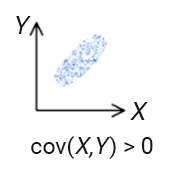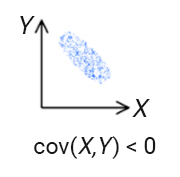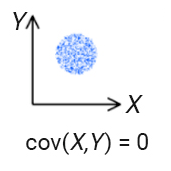## Covariance

Covariance is a measure of the joint variability of two or more random variables. If larger values of one variable correspond to larger values of another variable, and the same holds for smaller values (variables tend to show similar behavior), the covariance is positive.In the opposite case, when larger values of one variable correspond to smaller values of another variable (variables tend to show opposite behavior), the covariance is negative.If the values in one variable do not predict the values in another variable, the covariance is zero.The sign of covariance shows the tendency of linear relationship between the variables. However, the magnitude of the covariance is not easy to interpret as it is not normalized and depends on the magnitude of the variables. The normalized version of the covariance, the correlation coefficient, shows the strength of the linear relation by its magnitude.

The sample covariance of two samples$X$ and$Y$ are:$cov(X,Y)=E(X-E(X))E(Y-E(Y))=\frac{1}{N-1}\sum_{i=1}^{N}{(X_{i}-\bar{X})(Y_{i}-\bar{Y})}$

We use$N-1$ instead of$N$ to make the estimator unbiased as we use the sample mean ($\bar(x)$ and$\bar(y)$) instead of the population mean in the computation. If the population mean is known, the unbiased estimator is:$cov(X,Y)=\frac{1}{N}\sum_{i=1}^{N}{(X_{i}-E(X))(Y_{i}-E(Y))}$